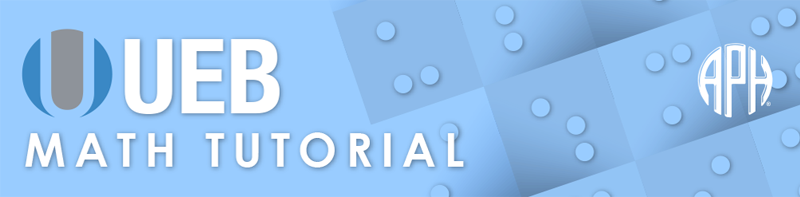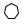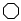Practice Problems -   -  Use 6 Dot Entry   Switch to Nemeth Tutorial

# Lesson 4.4: Shapes

## Symbols

$\text{shape indicator}$

$\text{filled shape indicator}$
⠸⠫

$\text{shaded shape indicator}$
⠨⠫

$\text{shaded terminator}$

$\square \phantom{\rule{.3em}{0ex}}\text{square}$
⠫⠼⠙

$◯\phantom{\rule{.3em}{0ex}}\text{circle}$
⠫⠿

$△\phantom{\rule{.3em}{0ex}}\text{triangle}$
⠫⠼⠉

## Explanation

A shape in print can be used to represent its geometric form. The shape symbol should be used only when a corresponding miniature shape symbol is used in the printed matter. Shape symbols are also used to represent omitted items or place-value holders in mathematical expressions. The shape used in print should be used in braille.

The shape indicator is dots one two four six. The shape indicator is followed by a numeral or a configuration of dots to represent the geometric shape. Follow print for spacing and punctuation of the shape. When a shape represents an omission, the shape is spaced based on the omitted item it represents. The shape indicator has a grade 2 meaning, therefore it must be in grade 1 mode. The shape indicator turns on shape mode and no further grade 1 indicators are needed for the shape. Shape mode is terminated by a space or the shape terminator.

Print shape symbols can be hollow, shaded, or filled. The filled shape indicator is formed with two cells, dots four five six in the first cell and dots one two four six in the second cell. The shaded shape indicator is formed with dots four six in the first cell and dots one two four six in the second cell. The filled and shaded shape indicators do not require grade 1 mode as they do not have a grade 2 meaning. The shape terminator, dots one five six, is required when the shape is not immediately followed by a space.

### Example 1

$△\phantom{\rule{.3em}{0ex}}\text{}ABC$
⠰⠫⠼⠉⠀⠠⠠⠁⠃⠉

### Example 2

$▴\phantom{\rule{.3em}{0ex}}\text{}DEF$
⠸⠫⠼⠉⠀⠠⠠⠙⠑⠋

### Example 3

$6×4=\square$
⠼⠋⠐⠦⠼⠙⠀⠐⠶⠀⠰⠫⠼⠙

In Examples 4 and 5, grade 1 mode is initiated by the numeric indicator at the beginning of the expression, therefore a grade 1 indicator is not needed. Example 4 illustrates a circle shape used to indicate an omitted sign of operation and in Example 5 the triangle shape is used to represent a missing numeral in an addition problem.

### Example 4

$12◯4=3$
⠼⠁⠃⠫⠿⠱⠼⠙⠀⠐⠶⠀⠼⠉

### Example 5

$3+△=5$
⠼⠉⠐⠖⠫⠼⠉⠀⠐⠶⠀⠼⠑

The grade 1 indicator is required with the shape indicator in Example 6 because the shape occurs at the beginning of the expression.

### Example 6

$\square -7=23$
⠰⠫⠼⠙⠱⠐⠤⠼⠛⠀⠐⠶⠀⠼⠃⠉

### Example 7

$\text{A}\phantom{\rule{.3em}{0ex}}△\phantom{\rule{.3em}{0ex}}\text{has three sides.}$
⠠⠁⠀⠰⠫⠼⠉⠀⠓⠁⠎⠀⠹⠗⠑⠑⠀⠎⠊⠙⠑⠎⠲

### Example 8

⠠⠗⠑⠏⠇⠁⠉⠑⠀⠮⠀⠰⠫⠿⠀⠕⠗⠀⠰⠫⠼⠙⠀⠾⠀⠈⠣⠀⠕⠗⠀⠈⠜⠲

### Example 9

$△ABC$
⠰⠫⠼⠉⠱⠠⠠⠁⠃⠉

### Example 10

$◯,\phantom{\rule{.3em}{0ex}}\square ,\phantom{\rule{.3em}{0ex}}△$
⠰⠫⠿⠱⠂⠀⠰⠫⠼⠙⠱⠂⠀⠰⠫⠼⠉

### Example 11

$\text{Are}\phantom{\rule{.3em}{0ex}}△\text{'s}\phantom{\rule{.3em}{0ex}}A\phantom{\rule{.3em}{0ex}}\text{and}\phantom{\rule{.3em}{0ex}}B\phantom{\rule{.3em}{0ex}}\text{acute?}$
⠠⠜⠑⠀⠰⠫⠼⠉⠱⠄⠎⠀⠠⠁⠀⠯⠀⠰⠠⠃⠀⠁⠉⠥⠞⠑⠦

## More Shapes

$⬠\phantom{\rule{.3em}{0ex}}\text{regular pentagon}$
⠫⠼⠑

$⬡\phantom{\rule{.3em}{0ex}}\text{regular hexagon}$
⠫⠼⠋$\phantom{\rule{.3em}{0ex}}\text{regular heptagon}$
⠫⠼⠛$\phantom{\rule{.3em}{0ex}}\text{regular octagon}$
⠫⠼⠓

$▱\phantom{\rule{.3em}{0ex}}\text{parallelogram}$
⠫⠈⠼⠙